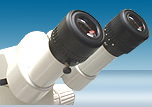Home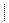About UsAnalytical TechniquesProblem SolvingAnalysisConsultancyIndustrial SectorsContact UsAnalytical TechniquesCoefficient of Friction MeasurementMechanical PropertiesMechanical Tensile TestingFriction MeasurementHardness TestingMetallographic AnalysisOptical Microscopy and Image AnalysisScanning Electron Microscopy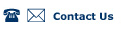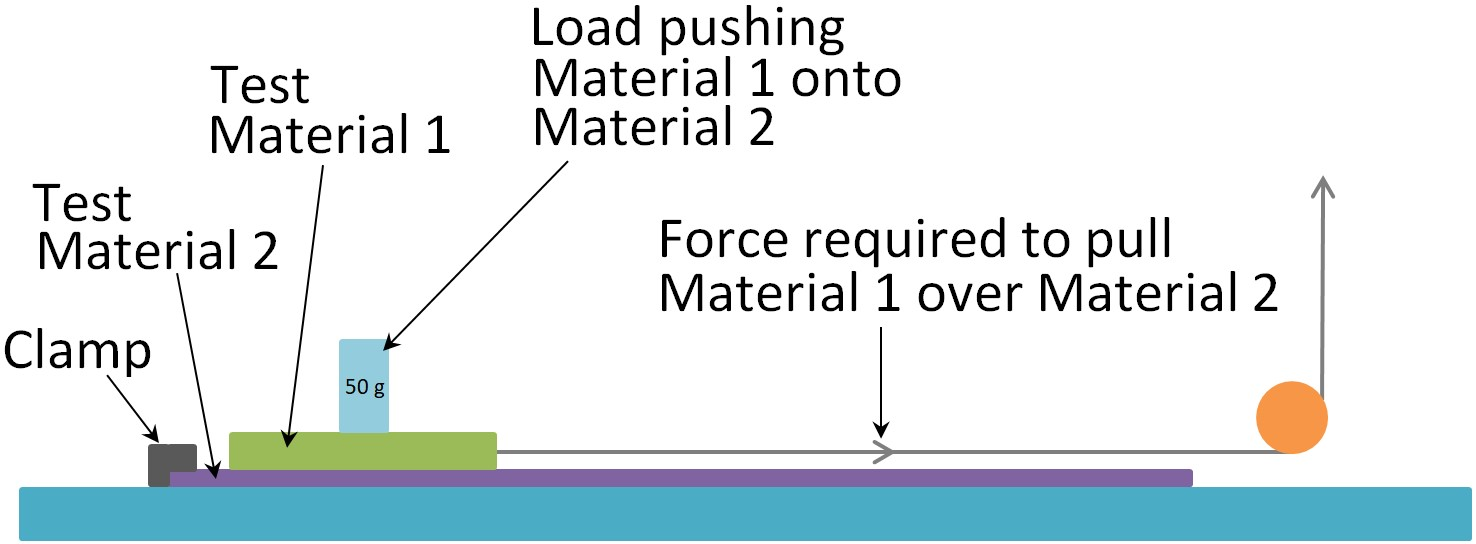Friction is a frequently overlooked phenomenon that plays an important role in our daily lives making friction measurments important.  Friction requires consideration in all industries: mining, manufacturing, transport, textiles and sport, to name a few. For certain applications it may be very important to have a high coefficient of friction, for example to prevent slipping of packaged items that need to be stacked high – a safety issue. Sometimes a low coefficient of friction is required, for example where production items need to slide down a chute to the next process – a logistics issue.

LPD Lab Services possesses the skill and equipment to determine the coefficient of friction of your materials. If you are buying materials, the information from friction tests will help you select materials with the required frictional properties. If you are manufacturing materials, then friction testing can help you find the optimum process adjustments for your product to meet you clients’ needs where friction is a decision factor.Friction testing is performed according to a standard procedure, such as ISO 8295 or ASTM D1894, using our Testometric M500-50CT tensile test machine with either a 50N, 2.5kN or 50kN load cell, depending on the weight of the test samples. The test samples are typically prepared such that both have at least one flat surface. One surface is held static whilst the other is pulled over the static surface. The following image shows a typical test setup.

Friction is the force that counteracts relative movement between two touching surfaces and the coefficient of friction (µ) is the ratio between the force required to pull one surface over the other (f) and the force by which the surfaces are pressed against each other (N).

μ= f/N

The coefficient of friction between two surfaces remains constant as long as the surface conditions remain the same. It does not change if the load between the two surfaces, the surface areas or the speed of movement changes. The following graph is a plot of the force required to move one surface over the other as the force pushing one surface against the other is changed. The slope of the graph is equal to the coefficient of friction.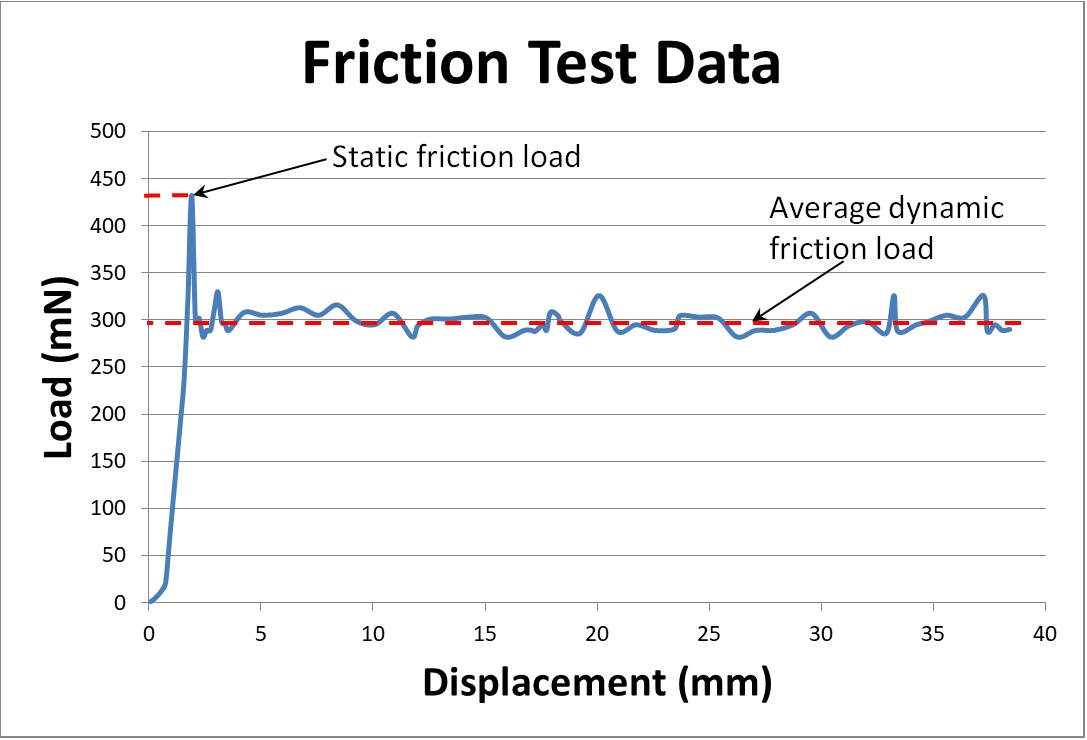There are two kinds of friction, static and kinetic. Static friction is the force preventing an object from moving when it is static. The static coefficient of friction is calculated using the maximum force required to induce movement between the two surfaces. Kinetic friction is the force opposing movement between two surfaces, when it is in movement. The kinetic coefficient of friction is calculated using the average force required to move the one surface over the other. The following plot is generated during the friction test and shows how the force required to move one surface over the other changes as it is displaced at a constant rate.

Our Experts are Dr Wyndham Johnstone and Danie Els. Contact us if you have any questions on friction measurements.Copyright 2021 LPD Lab Services Ltd. All rights reserved.